Blackjack
 Home | Gambling | Software | Articles | Books

Blackjack is a card game that is actually symmetric for the players and the house (dealer) and one of few casino games allowing an optimal play that reduces the house edge. Obtaining such a strategy was not an easy task for the mathematicians dealing with the game. The gaming events are formulated as referring to sums of values and not specific combinations of cards of a constant size. Any relevant probability for the gaming events in blackjack is conditional: Calculating the probability that a player will win the hand at a certain moment depends upon the future cards of the dealer and changes with every card dealt. Moreover, this probability alone cannot stand as the only criterion for a strategy, but only compared with the conditional probabilities that the dealer will beat the player’s hand or go bust.

Modern blackjack is not very often played with one complete deck of cards as it was in its early times; instead, it is played with a stack coming from several decks (usually six), shuffled and divided off by a blank card at about one-fifth of the pack. This rule of course prevents knowing the exact probabilities of occurrence of the various values of cards as the cards are dealt, since they are not evenly distributed in the composition of the stack in play.

For the games played with complete decks of cards, we first present the probabilities associated to card dealing and initial predictions. The probabilities below are calculated for games using one or two decks of cards. Let us look at the probabilities for a favorable initial hand (the first two cards dealt) to be achieved. The total number of possible combinations for each of the two cards is C(52, 2) = 1326, for 1-deck game and C(104, 2)=5356 for 2-deck game.

Probability of obtaining a natural blackjack is  P = 8/663 = 1.20663%  in the case of a 1-deck game and  P = 16/1339 = 1.19492%  in the case of a 2-deck game.

Probability of obtaining a blackjack from the first two cards is  P = 32/663 = 4.82654%  in the case of a 1-deck game and  P = 64/1339= 4.77968%  in the case of a 2-deck game.

Similarly, we can calculate the following probabilities:

Probability of obtaining 20 points from the first two cards is  P = 68/663 = 10.25641%  in the case of a 1-deck game and  P = 140/1339 = 10.45556%  in the case of a 2-deck game.

Probability of obtaining 19 points from the first two cards is  P = 40/663 = 6.03318%  in the case of a 1-deck game and  P = 80/1339 = 5.97460%  in the case of a 2-deck game.

Probability of obtaining 18 points from the first two cards is  P = 43/663 = 6.48567%  in the case of a 1-deck game and  P = 87/1339 = 6.4973%  in the case of a 2-deck game.

Probability of getting 17 points from the first two cards is  P = 16/221 = 7.23981%  in the case of a 1-deck game and  P = 96/1339 = 7.16952%  in the case of a 2-deck game.

A good initial hand (which you can stay with) could be a blackjack or a hand of 20, 19 or 18 points. The probability of obtaining such a hand is calculated by totaling the corresponding probabilities calculated above:  P = 32/663 + 68/663 + 40/663 + 43/663 = 183/663, in the case of a 1-deck game and  P = 64/1339 + 140/1339 + 80/1339 + 87/1339 = 371/1339, in the case of a 2-deck game.

Probability of obtaining a good initial hand is  P = 183/663 = 27.60180%  in the case of a 1-deck game and  P = 371/1339 = 27.70724%  in the case of a 2-deck game.

The probabilities of events predicted during the game are calculated on the basis of the played cards (the cards showing) from a certain moment. This requires counting certain favorable cards showing for the dealer and for the other players, as well as in your own hand. Unlike a baccarat game, where a maximum of three cards are played for each player, at blackjack many cards could be played at a certain moment, especially when many players are at the table. Thus, both following and memorizing certain cards require some ability and prior training on the player’s part.

If the game is played with complete decks of cards, the probability of occurrence of a certain value during the game would be given by a simple formula: If p(x) is the probability of a card with value x being dealt as the next card, m is the number of decks used, n(x) the number of x-valued cards already dealt (seen), and N the total number of cards already dealt, then:

P(x) = [4mn(x)]/(52mN)  if x is different from 10 and

P(x) = [16mn(x)]/(52mN)  if x is 10.

Example of application of the formula:  Assume you play with one deck, you are the only player at table, you hold Q, 2, 4, A (total value 17) and the face-up card of the dealer is a 4. Let us calculate the probability for you to achieve 21 points (receiving a 4) with the next card dealt.

We have m = 1, n(x) = 2, N = 5, so:.

For the probability of achieving 20 points (receiving a 3), we have  n(x) = 0, N = 5, so: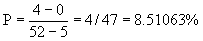.

For the probability of achieving 19 points (receiving a 2), we have  n(x) = 1, N = 5, so:.

If we want to calculate the probability of achieving 19, 20 or 21 points, we have to add together the three probabilities just calculated. We get  P = 9/47 = 19.14893%.

When playing with a random stack from several decks, such computation is not possible, as we do not know the total number of cards of value x are in the stack. In regard to developing an optimal strategy in blackjack, this premise imposed a mathematical approach based on approximations, through which to by-pass the difficulty of probability computations while not affecting in any way the optimal character of the strategy. This was the task of several mathematicians in the 1950s, whose work shaped the first optimal strategy in blackjack. The basic idea was to assign constant probabilities to the individual card values, namely 1/13 for the cards with value different from 10 and 4/13 for those with value 10, regardless of the cards dealt in the progress of the game. This approximation and supposition is of course adequate only for a stack of cards of infinite size since the probabilities change as the cards are dealt. However, the intended fixed strategy will eventually approximate the optimal strategy on average.

Optimal fixed strategy

The driving principle was that a good strategy should be oriented to the dealer’s first card as carrying significant information about the course that the dealer’s hand will likely take. The end probability distribution for the dealer is shown in the next table:

Result:               17       18      19       20       21    blackjack   bust

Probability:      0.145  0.139  0.133  0.180  0.072     0.047     0.281

The dealer will go bust with a 28.1% probability, or in frequential terms, more than one in every four hands on average.

A player who copies the house’s strategy, without splitting or doubling, until at least 17 points are achieved will have the same probabilities as those in the table above. If the game’s rules were the same for the house as for the player, then the player’s expectation for a bet would be zero. But this is not the case and a player who follows the house’s strategy has actually an expectation of minus 5.68% of the stake (a loss). In deciding how to play, a player should use the information given by the dealer’s first card and compare the profit/loss expectations (conditioned on the dealer’s first card and player’s current value in hand) in the possible choices – drawing or not drawing another card, doubling or not doubling, and splitting or not splitting. The higher expectation indicates the optimal choice.

The applicable results of the fixed optimal strategy are shown in the following tables of values of the expectation, all of which have the dealer’s first card and player’s total value as their row-column variables (inputs). The optimal drawing strategy works in the reverse direction of the progress of the game; that is, the player begins with the high totals and then optimizes the strategy recursively step by step down to lower values with the aid of the expectation tables.Table1. Probabilities for the dealer's results conditioned on the dealer's first card.Table 2. Expectations, conditioned on the dealer's first card, for the player's profit, when the player stays.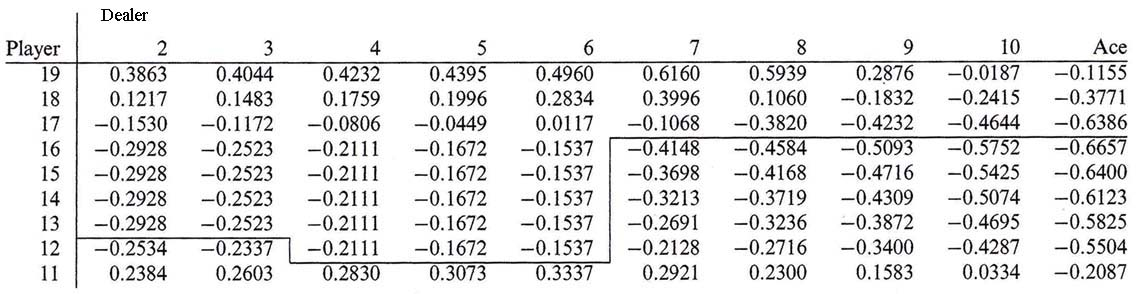Table 3. Expectations, conditioned on the dealer's first card, for the player's profit, when the player draws.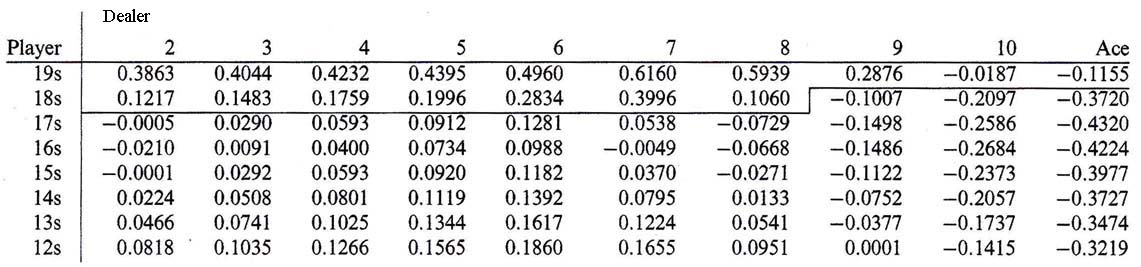Table 4. Expectations, conditioned on the dealer's first card, for the player's profit, when the player draws from a soft hand.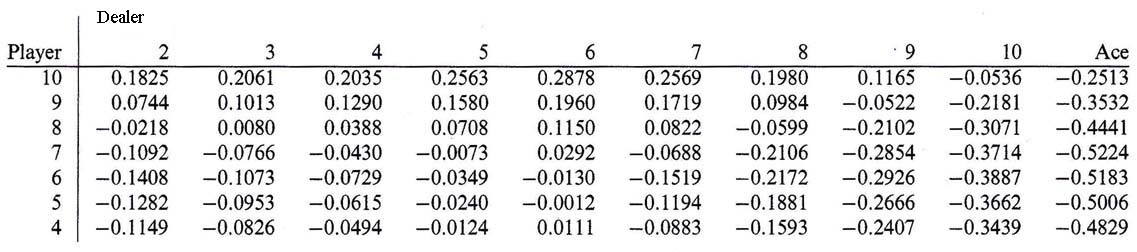Table 5. Expectations, conditioned on the dealer's first card, for the player's profit, when the player plays optimally.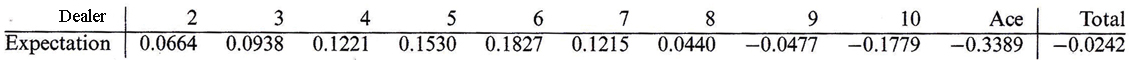Table 6. Expectations, conditioned on the dealer's first card, for the player's profit, when the player plays optimally without doubling or splitting.Table 7. Expectations, conditioned on the dealer's first card, for the player's profit, when the player draws exactly one more card.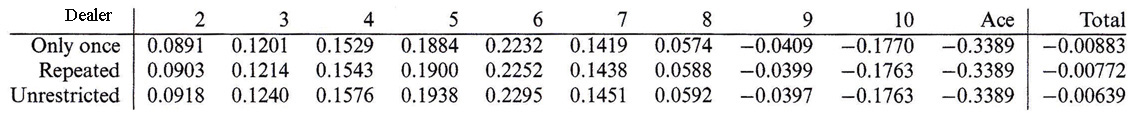Table 8. Expectations, conditioned on the dealer's first card, for the player's profit, for optimal play, depending on the splitting rule.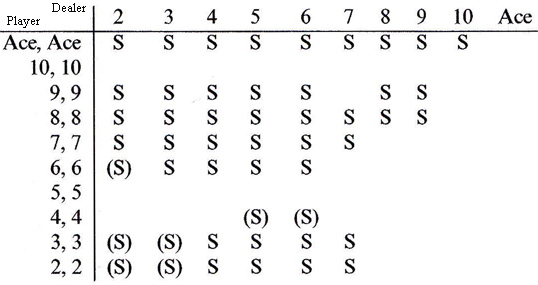Table 9. When splitting is advantageous; S indicates that splitting is advantageous, while (S) indicates that splitting is advantageous only if the next card is allowed to be doubled.

From the tables one can see that such strategy is relatively defensive. For instance:

– Against the dealer’s first card 4, 5, or 6, the player should draw only to 11.

– Against the dealer’s first card 2 or 3, the player should go to 13 and above.

– Against the dealer’s first card from 7 to ace, the player should draw to 17.

Depending on the set of rules, with the optimal play described above, the average loss can be brought down between 0.64% and 0.88% of the initial wager (less than the average loss for the simple bets in roulette, which is 1.35%). The optimal play based on comparison of mathematical expectations did not employ the information available for the player about the cards already played (viewed) and does not imply changes in strategy with the cards drawn, but only has totals as inputs; this is why it is called the optimal fixed strategy.

High-low-count optimal strategy

In the 1960s mathematician Edward Thorp investigated more extensively whether the information available about the cards already played could significantly increase the player’s winning expectation and how it could be quantified for use in an optimal strategy. The basic idea was to assign a weight to each card value played, as follows: +1 for 2 through 6; –1 for 10, face cards, and ace; 0 for the remaining cards. This turned out to be a practical counting system based on an approximation, whose total at a certain moment (called the count) quantified sufficiently well the information given by the cards played for being transformed into a mathematical criterion for changing the strategy according to the count. It was called the high-low system. The high-low counting system, although approximal in reflecting the situation of the cards played, was proved by Thorp to reflect exactly the change in winning expectation.

The criterion driving the optimal strategy is the value of the fraction C/n, where C is the result of the count and n the number of remaining cards in the deck/stack. The strategies of drawing, doubling and splitting are expressed in tables of values for 100C/n, where the input variables are dealer’s first card and player’s total in hand:

Bet:   If 100C/n ≥ 3.6, then raise your bet; otherwise, keep your bet at minimum.

Drawing:

Draw at "D" or when 100C/n is less than or equal to the given value:Table 10.

Draw at "D" or when 100C/n is less than or equal to the given value:Table 11.

Doubling:

Double when 100C/n is greater than or equal to the given value: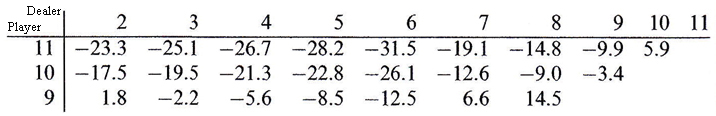Table 12.

Splitting:

Split on "S" or when 100C/n is greater than or equal to the given value (The table does not allow for split hands to be doubled):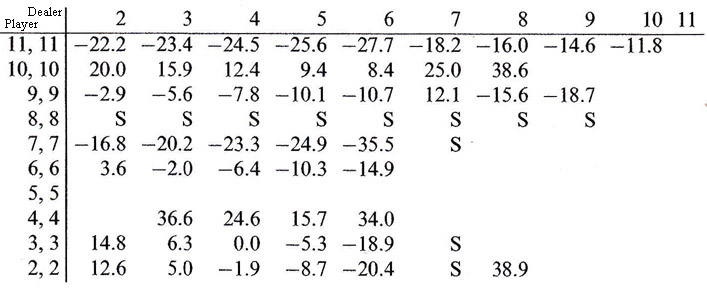Table 13.

 Author The author of this page is Catalin Barboianu (PhD). Catalin is a games mathematician and problem gambling researcher, science writer and consultant for the mathematical aspects of gambling for the gaming industry and problem-gambling institutions. Profiles:   Linkedin   Google Scholar   ResearchgateCheck this
 Do you own a gambling website and want backlinks from high-rank academic websites? Click here. New release! Understanding Your Game: A Mathematician's Advice for Rational and Safe GamblingClick here.